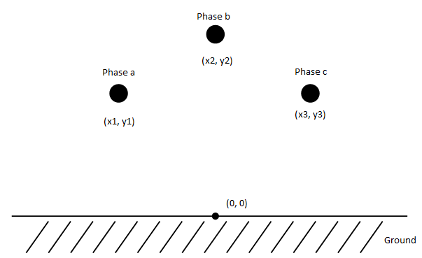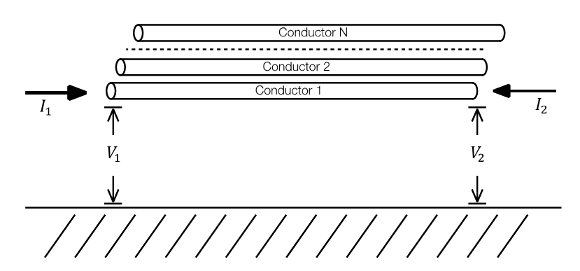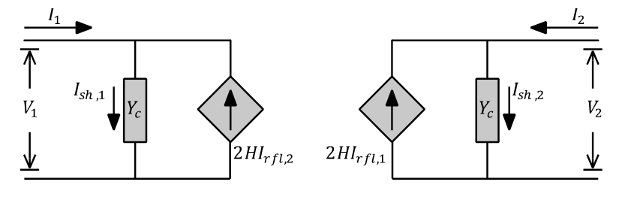# Frequency-Dependent Overhead Line (Three-Phase)

Three-phase overhead line which includes effects that vary as a function of frequency

• Library:
• Simscape / Electrical / Passive / Lines

•## Description

The Frequency-Dependent Overhead Line (Three-Phase) block represents a high-fidelity frequency-dependent overhead line that offers accurate transient response simulation from 0.01 Hz to 100 kHz.

The block computes frequency-dependent impedance and admittance matrices. The calculations also depend on the loss, inductance, and capacitance of the return path.

For more information on using a frequency-dependent overhead line (three-phase), see Engineering Applications.### Equations

Line ModelThe electromagnetic behavior of a multiconductor transmission line is described by the telegrapher's equation.

`$-\frac{dV}{dx}=ZI$`

`$-\frac{dI}{dx}=YV$`

Where:

• V is the vector of line phase voltages.

• I is the vector of phase currents.

• Z is the series impedance matrix in per unit length.

• Y is the shunt admittance matrix in per unit length.

These are general solutions for the vector of currents and voltages.

 $I\left(x\right)={e}^{-\text{Ψ}x}{C}_{1}+{e}^{\text{Ψ}x}{C}_{2}$ (1)

 (2)

Where $\text{Ψ}=\sqrt{YZ}$ is the propagation matrix and ${Y}_{c}={\left(\sqrt{YZ}\right)}^{-1}Y$ is the characteristic admittance.

Consider now a transmission line segment of length x = l. At the beginning of one of its ends, when x = 0, the equations 1 and 2 are evaluated as follows.

 ${I}_{1}={C}_{1}+{C}_{2}$ (3)

 (4)

The integration constant vectors C1 and C2 can be expressed in terms of I0 and V0.

 ${C}_{1}=\frac{\left({I}_{1}+{Y}_{c}{V}_{1}\right)}{2}$ (5)

 ${C}_{2}=\frac{\left({I}_{1}-{Y}_{c}{V}_{1}\right)}{2}$ (6)

Similarly, at x = l, equations 1 and 2 are evaluated as follows.

 ${I}_{2}={e}^{-\Psi \text{l}}{C}_{1}+{e}^{\Psi \text{l}}{C}_{2}$ (7)

 ${Y}_{c}{V}_{2}={e}^{-\Psi \text{l}}{C}_{1}-{e}^{\Psi \text{l}}{C}_{2}$ (8)

To obtain the two fundamental equations for the transmission line model, first you use equations 7 and 8 to perform this calculation.

 ${I}_{2}-{Y}_{c}{V}_{2}=-2{e}^{-\Psi l}{C}_{1}$ (9)

Then you substitute equation 5 into equation 9.

 ${I}_{2}-{Y}_{c}{V}_{2}=-H\left({I}_{1}+{Y}_{c}{V}_{1}\right)$ (10)

Where $H={e}^{-\text{Ψ}l}$ is the propagation factor matrix. Equation 10 establishes the relation between voltages and currents at the terminals of a multi-conductor line section.

You can obtain a companion expression providing the model for the terminal 1 at x = 0.

 ${I}_{1}-{Y}_{c}{V}_{1}=-H\left({I}_{2}+{Y}_{c}{V}_{2}\right)$ (11)

Define:

• ${I}_{sh,1}={Y}_{c}{V}_{1}$ — Shunt current vector produced at terminal 1 by injected voltages V1

• ${I}_{sh,2}={Y}_{c}{V}_{2}$ — Shunt current vector produced at terminal 2 by injected voltages V2

• ${I}_{rfl,1}=\frac{1}{2}\left({I}_{1}+{Y}_{c}{V}_{1}\right)$ — Reflected currents of terminal 1

• ${I}_{rfl,2}=\frac{1}{2}\left({I}_{2}+{Y}_{c}{V}_{2}\right)$ — Reflected currents of terminal 2

And finally rewrite equations 10 and 11 as follows:

`${I}_{1}={I}_{sh,1}-2H{I}_{rfl,2}$`

`${I}_{2}={I}_{sh,2}-2H{I}_{rfl,1}$`

These equations constitute a traveling wave line model for the segment of length L.Particularly for transmission lines with ground return, the parameters are highly dependent on frequency. Model solutions are then carried out directly in the phase domain. The block computes automatically the impedance and the characteristic admittance matrices on the full frequency range, performing an approximation through rational fitting.

Rational fitting for this model is performed by using the vector fitting (VF) procedure. For more information on the phase domain line model and the state-space analysis, see Wide-Band Line Model Implementation in Matlab for EMT Analysis .

### Variables

To set the priority and initial target values for the block variables prior to simulation, use the Initial Targets section in the block dialog box or Property Inspector. For more information, see Set Priority and Initial Target for Block Variables.

Nominal values provide a way to specify the expected magnitude of a variable in a model. Using system scaling based on nominal values increases the simulation robustness. Nominal values can come from different sources, one of which is the Nominal Values section in the block dialog box or Property Inspector. For more information, see System Scaling by Nominal Values.

## Ports

### Conserving

expand all

Expandable electrical conserving three-phase port associated with AC voltage 1.

Expandable electrical conserving three-phase port associated with AC voltage 2.

## Parameters

expand all

Whether to model composite or expanded three-phase ports.

Composite three-phase ports represent three individual electrical conserving ports with a single block port. You can use composite three-phase ports to build models that correspond to single-line diagrams of three-phase electrical systems.

Expanded three-phase ports represent the individual phases of a three-phase system using three separate electrical conserving ports.

Position of the line in terms of the x-axis of the reference frame.

Position of the line in terms of the y-axis of the reference frame. The elements of the vector need to be greater than `0`.

Conductor resistivity. The value must be greater than `0`.

Earth return resistivity. The value must be greater than `0`.

Conductor radius. The value must be greater than `0`.

Line length. The value must be greater or equal than `1` and less or equal than `3e5`.

Configuration of the ground nodes.